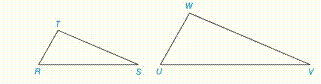Chapter 5.CR, Problem 16CRElementary Geometry For College St...

7th Edition
Alexander + 2 others
ISBN: 9781337614085

Solutions

Chapter
SectionElementary Geometry For College St...

7th Edition
Alexander + 2 others
ISBN: 9781337614085
Textbook Problem

Name the method (AA, SSS˜, or SAS˜) that is used to show that the triangles are simiar.Use the figure below. a) W U = 2 ⋅ T R , W V = 2 ⋅ T S ,       and       U V = 2 ⋅ R S   b) ∠ T ≅ ∠ W     and       ∠ S ≅ ∠ V c) ∠ T ≅ ∠ W       a n d     T R W U = T S W V d)     T R W U = T S W V = R S U VTo determine

a)

To name:

Method used to show the triangles are similar.

Explanation

Given:

WU=2TR,WV=2TS and UV=2RS

Calculation:

From the triangle, ΔTRS and ΔWUV

The length of WU=2TR,WV=2TS and UV=2RS

That is,

To determine

b)

To name:

Method used to show the triangles are similar.

To determine

c)

To name:

Method used to show the triangles are similar.

To determine

d)

To name:

Method used to show the triangles are similar.

Still sussing out bartleby?

Check out a sample textbook solution.

See a sample solution

The Solution to Your Study Problems

Bartleby provides explanations to thousands of textbook problems written by our experts, many with advanced degrees!

Get Started

Find f. f(t)=t2cost

Single Variable Calculus: Early Transcendentals

For and

Study Guide for Stewart's Multivariable Calculus, 8th

For y = x sin x, y = _____. a) x cos x b) x cos x + 1 c) cos x d) x cos x + sin x

Study Guide for Stewart's Single Variable Calculus: Early Transcendentals, 8th